### Rate Equation from CSTR data

From the following data find a satisfactory rate equation for the gas-phase decomposition of pure A, A -> R + S, in a mixed flow reactor.

 τ based on inlet feed conditions, sec 0.423 5.1 13.5 44 192 XA (for CAo = 0.002 mol/lit) 0.22 0.63 0.75 0.88 0.96

Calculations:

For the mixed flow reactor, the design equation is,

τ = CAoXA/-rA → 1

For variable density systems,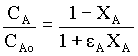Where, εA = fractional change in volume = (VXA = 1 - VXA = 0) / VXA = 0 = (2 - 1)/1 = 1

Therefore,à 2

For the first order equation, equn.1 can be written as

τ = CAoXA/ kCA → 3

substituting for CAo in equation 3,

τ = 0.002 XA/ kCA

substituting for CA from equn.2,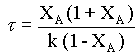That is, if the given data is for a first order reaction, τ vs. XA(1 + XA)/(1 - XA) will be a straight line, with a slope of k.

 τ, sec 0.423 5.1 13.5 44 192 XA 0.22 0.63 0.75 0.88 0.96 XA(1 + XA)/(1 - XA) 0.3441 2.7754 5.25 13.7867 47.04

From the above data, the following graph is drawn :(graph 1)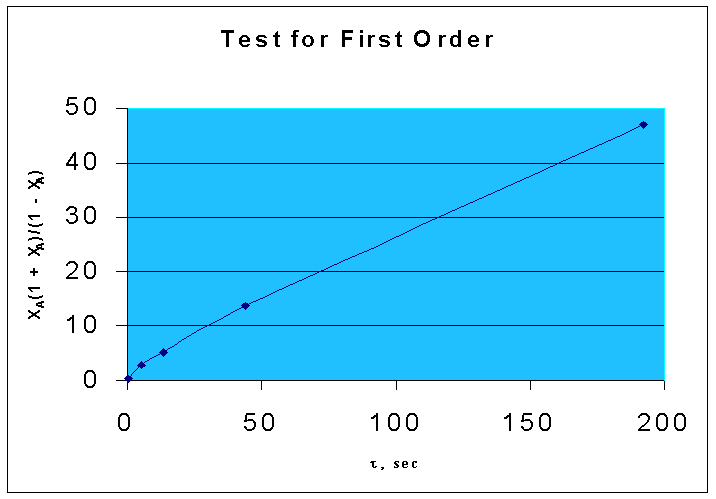For the second order equation, equn.1 can be written as

τ = CAoXA/ kCA2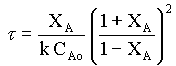That is, if the given data is for a second order reaction, τ vs. XA(1 + XA)2/ (1 - XA)2 will be a straight line with a slope of kCAo.

 τ, sec 0.423 5.1 13.5 44 192 XA 0.22 0.63 0.75 0.88 0.96 XA(1 + XA)2/(1 - XA)2 0.5382 12.2268 36.75 215.99 2304.96

From the above data, the following graph is drawn :(graph 2)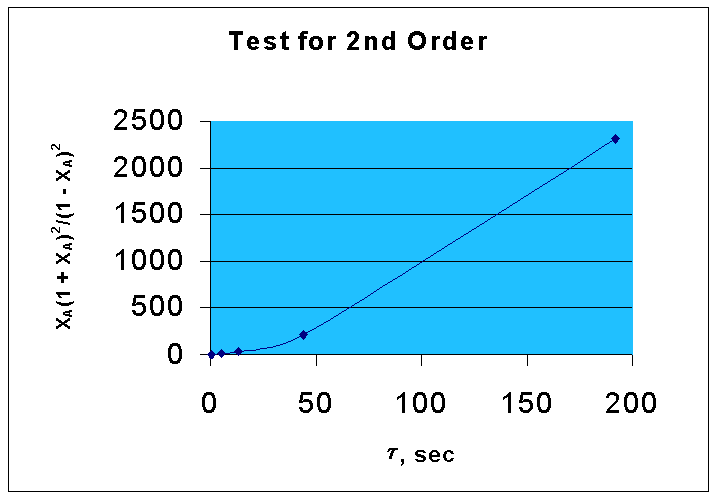By comparing two graphs it is seen that first order kinetics is well fitting the given data.

That is, the reaction is following first order.

Slope = 0.2493 (from the graph.1)

Therefore,

k = 0.2493 sec-1.

Therefore, rate equation for the given reaction is

-rA = 0.2493 CAo (1 - XA)/(1 + XA) = 4.986 x 10-4 (1 - XA)/(1 + XA) mol/lit.sec